java 判断两个字符串是否为相同字母异序词

fkg 发布于 2019年05月15日 (共有20个回帖)

String org1=“arm”; String org2="ram"; return true;

String org3="des"; String org4="dec"; return false;

public static boolean compareArg(String a,String b){
if(a.length()!=b.length()){
return false;
}
a=a.toLowerCase();
b=b.toLowerCase();
int temp=0;
for(int j=0;j<b.length();j++){
String c=b.substring(j,j+1);
if(a.contains(b.substring(j,j+1))){
temp=temp+1;
}
}
if(a.length()==temp){
return true;
}
return false;
}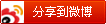• 网友回帖 (20)
•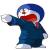loyal 发表于 5个月前 如果有重复,你这个方法就有问题了吧? abcc cabc abc
•loyal 发表于 5个月前 你的temp没啥意义...还不如没有时直接return false;
•loyal 发表于 5个月前 还有一种方式,叫逻辑简单,但代码上比较长. 1.先将两个字符串排序. 2.最后一个一个对应着比较. --- 共有 1 条评论 --- fkg(5个月前)：代码虽长，但是是万无一失的方法。
•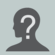SapphireR 发表于 5个月前 你没考虑重复的情况，略改了下 public static boolean compareArg(String a, String b) { if (a.length() != b.length()) { return false; } a = a.toLowerCase(); b = b.toLowerCase(); char[] ac = a.toCharArray(); char[] bc = b.toCharArray(); Arrays.sort(ac); Arrays.sort(bc); a = String.valueOf(ac); b = String.valueOf(bc); return a.equals(b); } --- 共有 1 条评论 --- fkg(5个月前)：不错，学习啦~~~
•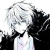DrakKing 发表于 5个月前 可以两个字符串按asc排序在判断是否相等。
•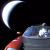Junior_s 发表于 5个月前 先判断是否相等（异序），然后排序后判断是否相等（同位）
•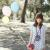fkg 发表于 5个月前 根据各位大佬的回复，对上述代码做了修正： public static boolean compareArg(String a,String b){ if(a.length()!=b.length()){ return false; } a=a.toLowerCase(); char[] as=a.toCharArray(); Arrays.sort(as); b=b.toLowerCase(); char[] bs=b.toCharArray(); Arrays.sort(bs); for(int j=0;j
•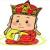前端大师傅 发表于 5个月前 这个很容易呀，在下有一个思路可以给楼上几位朋友扩展一下，和几位的思想完全不一样。 打个比方： 如果 A代表一个苹果，B代表两个苹果，C代表三个苹果，依次类推，那按楼主的说法如果 说字母的内容相同，只是顺序不同的话，那他们的和都是一样的。 即ABC,BCA,ACB他们的和肯定相同，而ACD,ACE,只有一个字母不同他们的和肯定不同。由这个原理我们可以这样来实现： 不好意思，上次答案给错了，修改了一下代码逻辑，正确的应该是把要比较的集合先按字符索引排序，下面是改的代码： public bool CompareStrAB(string a, string b) { bool result = false; List keys = null; List aList = null, bList = null; try { keys = new List("a,b,c,d,e,f,g,h,i,j,k,l,m,n,o,p,q,r,s,t,u,v,w,x,y,z".Split(',')); //将字符串转为字符列表即"dsaf"=> {'d','s','a','f'}; aList = new List(a.ToArray()); bList = new List(b.ToArray()); //将每个字母所在的索引值进行排序，这样在相同的排序下如果只是序错了就可以相等 aList.Sort((aItem, bItem) => ((keys.IndexOf(string.Format("{0}", aItem)) < keys.IndexOf(string.Format("{0}", bItem)) ? -1 : (keys.IndexOf(string.Format("{0}", aItem)) > keys.IndexOf(string.Format("{0}", bItem)) ? 1 : 0)))); bList.Sort((aItem, bItem) => ((keys.IndexOf(string.Format("{0}", aItem)) < keys.IndexOf(string.Format("{0}", bItem)) ? -1 : (keys.IndexOf(string.Format("{0}", aItem)) > keys.IndexOf(string.Format("{0}", bItem)) ? 1 : 0)))); //如果两个字符串的索引和相等表明他们的字符值是相等只是有可能顺序不对 result = string.Join("", (from v in aList select string.Format("{0}", v)).ToList().ToArray()) == string.Join("", (from v in bList select string.Format("{0}", v)).ToList().ToArray()); } catch (Exception ex) { Console.WriteLine(ex.Message); } return result; } --- 共有 2 条评论 --- 前端大师傅(5个月前)：回复 @helplove : 你说的是对的，我改了一下，即对字符串先按字符顺序进行排序操作。即bdc=>索引为1，3，2，如果按索引排序可得到1，2，3，即bcd然后再对比就可以了。 helplove(5个月前)：和相同的一定就是同样的字母组合？a(0) + b(1) + f(5) == b(1) + c(2) + d(3) 这就不行了吧
•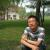光脚满地跑 发表于 5个月前 双排序的思路简单，复杂度略高 if(List1.size()!=List2.size) return false; else 是否可以采取打点的方式，遍历List2，判断每个元素在List中的位置，如果有则标记该位置（以使其不再被命中） 如果没有，则立即返回false
•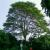风青山 发表于 5个月前 字符串是否为单词（中间没有空白符或其他特殊字符）的判断需要处理么？